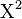# Chi-squared test facts for kids

Kids Encyclopedia Facts

Chi-squared test (or$\Chi^2$ test) is a statistical hypothesis test. It usually tests the hypothesis that "the experimental data does not differ from untreated data". That is a null hypothesis. The distribution of the test statistic is a chi-squared distribution when the null hypothesis is true.

The test results are regarded as 'significant' if there is only one chance in 20 that the result could be got by chance.

There are three main groups of tests:

• Tests for distribution check that the values follow a given probability distribution.
• Tests for independence check that the values are independent; if this is the case, no value can be left out without losing information.
• Tests for homogeneity: These check that all samples taken have the same probability distribution, or are from the same set of values.Chi-squared test Facts for Kids. Kiddle Encyclopedia.